Discrete Mathematics and Combinatorics Commons™

949 Full-Text Articles 1,167 Authors 104,022 Downloads95 Institutions

All Articles in Discrete Mathematics and Combinatorics

949 full-text articles. Page 7 of 35.

Facial Unique-Maximum Colorings Of Plane Graphs With Restriction On Big Vertices, 2018Iowa State University

Facial Unique-Maximum Colorings Of Plane Graphs With Restriction On Big Vertices, Bernard Lidicky, Kacy Messerschmidt, Riste Škrekovski

Mathematics Publications

A facial unique-maximum coloring of a plane graph is a proper coloring of the vertices using positive integers such that each face has a unique vertex that receives the maximum color in that face. Fabrici and Göring (2016) proposed a strengthening of the Four Color Theorem conjecturing that all plane graphs have a facial unique-maximum coloring using four colors. This conjecture has been disproven for general plane graphs and it was shown that five colors suffice. In this paper we show that plane graphs, where vertices of degree at least four induce a star forest, are facially unique-maximum 4-colorable. This ...

Templates For Representable Matroids, 2018Louisiana State University and Agricultural and Mechanical College

Templates For Representable Matroids, Kevin Manuel Grace

LSU Doctoral Dissertations

The matroid structure theory of Geelen, Gerards, and Whittle has led to a hypothesis that a highly connected member of a minor-closed class of matroids representable over a finite field is a mild modification (known as a perturbation) of a frame matroid, the dual of a frame matroid, or a matroid representable over a proper subfield. They introduced the notion of a template to describe these perturbations in more detail. In this dissertation, we determine these templates for various classes and use them to prove results about representability, extremal functions, and excluded minors.

Chapter 1 gives a brief introduction to ...

Average Mixing Matrix Of Trees, 2018University of Waterloo

Average Mixing Matrix Of Trees, Chris Godsil, Krystal Guo, John Sinkovic

Electronic Journal of Linear Algebra

The rank of the average mixing matrix of trees with all eigenvalues distinct, is investigated. The rank of the average mixing matrix of a tree on n vertices with n distinct eigenvalues is bounded above by ⌈n/2⌉. Computations on trees up to 20 vertices suggest that the rank attains this upper bound most of the time. An infinite family of trees whose average mixing matrices have ranks which are bounded away from this upper bound, is given. A lower bound on the rank of the average mixing matrix of a tree, is also given.

Two Results In Drawing Graphs On Surfaces, 2018Louisiana State University and Agricultural and Mechanical College

Two Results In Drawing Graphs On Surfaces, Joshua E. Fallon

LSU Doctoral Dissertations

In this work we present results on crossing-critical graphs drawn on non-planar surfaces and results on edge-hamiltonicity of graphs on the Klein bottle. We first give an infinite family of graphs that are 2-crossing-critical on the projective plane. Using this result, we construct 2-crossing-critical graphs for each non-orientable surface. Next, we use 2-amalgamations to construct 2-crossing-critical graphs for each orientable surface other than the sphere. Finally, we contribute to the pursuit of characterizing 4-connected graphs that embed on the Klein bottle and fail to be edge-hamiltonian. We show that known 4-connected counterexamples to edge-hamiltonicity on the Klein bottle are hamiltonian ...

$\Gamma'$-Realizability And Other Musings On Inverse Domination, 2018Dalton State College

$\Gamma'$-Realizability And Other Musings On Inverse Domination, John Asplund, Joe Chaffee, James M. Hammer Iii, Matt Noble

We introduce and study $\gamma'$-realizable sequences. For a finite, simple graph $G$ containing no isolated vertices, $I \subseteq V(G)$ is said to be an \emph{inverse dominating set} if $I$ dominates all of $G$ and $I$ is contained by the complement of some minimum dominating set $D$. Define a sequence of positive integers $(x_1, \ldots , x_n)$ to be \emph{$\gamma'$-realizable} if there exists a graph $G$ having exactly $n$ distinct minimum dominating sets $D_1, \ldots, D_n$ where for each $i \in \{1, \ldots, n\}$, the minimum size of an inverse dominating set in $V(G) \setminus D_i ... The Search For The Cyclic Sieving Phenomenon In Plane Partitions, 2018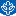DePaul University The Search For The Cyclic Sieving Phenomenon In Plane Partitions, William J. Asztalos DePaul Discoveries The efforts of this research project are best understood in the context of the subfield of dynamical combinatorics, in which one enumerates a set of combinatorial objects by defining some action to guide the search for underlying structures. While there are many examples with varying degrees of complexity, the necklace problem, which concerns the possible unique configurations of beads in a ring up to rotational symmetry, is a well-known example. Though this sort of approach to enumeration has been around for a century or more, activity in this area has intensified in the last couple of decades. Perhaps the most ... Additive List Coloring Of Planar Graphs With Given Girth, 2018Northern Kentucky University Additive List Coloring Of Planar Graphs With Given Girth, Axel Brandt, Sogol Jahanbekam, Jennifer Diemunsch Faculty Publications An additive coloring of a graph G is a labeling of the vertices of G from {1,2,...,k} such that two adjacent vertices have distinct sums of labels on their neighbors. The least integer k for which a graph G has an additive coloring is called the additive coloring number of G, denoted \luck(G). Additive coloring is also studied under the names lucky labeling and open distinguishing. In this paper, we improve the current bounds on the additive coloring number for particular classes of graphs by proving results for a list version of additive coloring. We apply the ... On The Distance And Distance Signless Laplacian Eigenvalues Of Graphs And The Smallest Gersgorin Disc, 2018Indian Institute of Technology Kharagpur On The Distance And Distance Signless Laplacian Eigenvalues Of Graphs And The Smallest Gersgorin Disc, Fouzul Atik, Pratima Panigrahi Electronic Journal of Linear Algebra The \emph{distance matrix} of a simple connected graph$G$is$D(G)=(d_{ij})$, where$d_{ij}$is the distance between the$i$th and$j$th vertices of$G$. The \emph{distance signless Laplacian matrix} of the graph$G$is$D_Q(G)=D(G)+Tr(G)$, where$Tr(G)$is a diagonal matrix whose$i$th diagonal entry is the transmission of the vertex$i$in$G$. In this paper, first, upper and lower bounds for the spectral radius of a nonnegative matrix are constructed. Applying this result, upper and lower bounds for the distance and distance signless ... Golden Arm: A Probabilistic Study Of Dice Control In Craps, 2018Monmouth University Golden Arm: A Probabilistic Study Of Dice Control In Craps, Donald R. Smith, Robert Scott Iii UNLV Gaming Research & Review Journal This paper calculates how much control a craps shooter must possess on dice outcomes to eliminate the house advantage. A golden arm is someone who has dice control (or a rhythm roller or dice influencer). There are various strategies for dice control in craps. We discuss several possibilities of dice control that would result in several different mathematical models of control. We do not assert whether dice control is possible or not (there is a lack of published evidence). However, after studying casino-legal methods described by dice-control advocates, we can see only one realistic mathematical model that describes the resulting ... On Coding For Partial Erasure Channels, 2018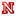University of Nebraska - Lincoln On Coding For Partial Erasure Channels, Carolyn Mayer Dissertations, Theses, and Student Research Papers in Mathematics Error correcting codes have been essential to the technology we use in everyday life in digital storage, wireless communication, barcodes, and much more. Different channel models are used for different types of communication (for example, if information is sent to one person or to many people) and different types of errors. Partial erasure channels were recently introduced to model applications in which some information remains after an erasure event. These remnants of information may be used to increase the chances of successful decoding. We introduce a new partial erasure channel in which partial erasures correspond to individual bit erasures in ... Graphs With Few Spanning Substructures, 2018University of Nebraska - Lincoln Graphs With Few Spanning Substructures, Jessica De Silva Dissertations, Theses, and Student Research Papers in Mathematics In this thesis, we investigate a number of problems related to spanning substructures of graphs. The first few chapters consider extremal problems related to the number of forest-like structures of a graph. We prove that one can find a threshold graph which contains the minimum number of spanning pseudoforests, as well as rooted spanning forests, amongst all graphs on n vertices and e edges. This has left the open question of exactly which threshold graphs have the minimum number of these spanning substructures. We make progress towards this question in particular cases of spanning pseudoforests. The final chapter takes on ... Vector Partitions, 2018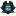East Tennessee State University Vector Partitions, Jennifer French Electronic Theses and Dissertations Integer partitions have been studied by many mathematicians over hundreds of years. Many identities exist between integer partitions, such as Euler’s discovery that every number has the same amount of partitions into distinct parts as into odd parts. These identities can be proven using methods such as conjugation or generating functions. Over the years, mathematicians have worked to expand partition identities to vectors. In 1963, M. S. Cheema proved that every vector has the same number of partitions into distinct vectors as into vectors with at least one component odd. This parallels Euler’s result for integer partitions. The ... The Doubly Stochastic Single Eigenvalue Problem: An Empirical Approach, 2018College of William & Mary The Doubly Stochastic Single Eigenvalue Problem: An Empirical Approach, John Wilkes, Charles Royal Johnson Undergraduate Honors Theses The doubly stochastic single eigenvalue problem asks what is the set DSn of all complex numbers that occur as an eigenvalue of an n-by-n doubly stochastic matrix. For n < 5, this set is known and for the analogous set for (singly) stochastic matrices, the set is known for all n. For Pk , the polygon formed by the k-th roots of unity, Unk=1 Pk ⊆ DSn, as is easily shown. For n < 5, this containment is an equality, but for n = 5, the containment is strict (though it is close). Presented here is substantial, computational evidence that the containment is an equality for 6 ≤ n ≤ 10 and for what DS5 actually is. Covering Arrays For Equivalence Classes Of Words, 2018East Tennessee State University Covering Arrays For Equivalence Classes Of Words, Joshua Cassels, Anant Godbole Undergraduate Honors Theses Covering arrays for words of length t over a d letter alphabet are k × n arrays with entries from the alphabet so that for each choice of t columns, each of the dt t-letter words appears at least once among the rows of the selected columns. We study two schemes in which all words are not considered to be different. In the first case, words are equivalent if they induce the same partition of a t element set. In the second case, words of the same weighted sum are equivalent. In both cases we produce logarithmic upper bounds on ... 2018Bowdoin College Exploring Random Walks On Graphs For Protein Function Prediction, Angela M. Dahl Honors Projects No abstract provided. The Average Measure Of A K-Dimensional Simplex In An N-Cube, 2018Missouri State University The Average Measure Of A K-Dimensional Simplex In An N-Cube, John A. Carter MSU Graduate Theses Within an n-dimensional unit cube, a number of k-dimensional simplices can be formed whose vertices are the vertices of the n-cube. In this thesis, we analyze the average measure of a k-simplex in the n-cube. We develop exact equations for the average measure when k = 1, 2, and 3. Then we generate data for these cases and conjecture that their averages appear to approach nk/2 times some constant. Using the convergence of Bernstein polynomials and a k-simplex Bernstein generalization, we prove the conjecture is true for the 1-simplex and 2-simplex cases. We then develop a generalized formula for ... 2018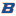Boise State University Secure Multiparty Protocol For Differentially-Private Data Release, Anthony Harris Boise State University Theses and Dissertations In the era where big data is the new norm, a higher emphasis has been placed on models which guarantees the release and exchange of data. The need for privacy-preserving data arose as more sophisticated data-mining techniques led to breaches of sensitive information. In this thesis, we present a secure multiparty protocol for the purpose of integrating multiple datasets simultaneously such that the contents of each dataset is not revealed to any of the data owners, and the contents of the integrated data do not compromise individual’s privacy. We utilize privacy by simulation to prove that the protocol is ... 2018William & Mary A New Upper Bound For The Diameter Of The Cayley Graph Of A Symmetric Group, Hangwei Zhuang Undergraduate Honors Theses Given a finite symmetric group S_n and a set S of generators, we can represent the group as a Cayley graph. The diameter of the Cayley graph is the largest distance from the identity to any other elements. We work on the conjecture that the diameter of the Cayley graph of a finite symmetric group S_n with S ={(12),(12...n)} is at most$ C(n,2). Our main result is to show that the diameter of the graph of S_n is at most (3n^2-4n)/2.

On Some Geometry Of Graphs, 2018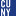The Graduate Center, City University of New York

On Some Geometry Of Graphs, Zachary S. Mcguirk

All Dissertations, Theses, and Capstone Projects

In this thesis we study the intrinsic geometry of graphs via the constants that appear in discretized partial differential equations associated to those graphs. By studying the behavior of a discretized version of Bochner's inequality for smooth manifolds at the cone point for a cone over the set of vertices of a graph, a lower bound for the internal energy of the underlying graph is obtained. This gives a new lower bound for the size of the first non-trivial eigenvalue of the graph Laplacian in terms of the curvature constant that appears at the cone point and the size ...

2018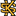Kennesaw State University

An Optimized Route For Q100'S Bert And Kristin To Visit All Jersey Mike's Subs In Atlanta For Charity

Symposium of Student Scholars

The Bert Show is a popular morning show on Atlanta’s Q100 radio station. They host a non-profit organization that provides a “magical, all-expenses-paid, five-day journey to Walt Disney World for children with chronic and terminal illnesses and their families” called “Bert’s Big Adventure.” On March 28th, 2018, thirty-seven locations of Jersey Mike’s are participating in the their Jersey Mike’s Day of Giving to support Bert’s Big Adventure. The goal is to have two popular radio show hosts visit each of these locations for some photos and presence to draw in more customers! But how ...